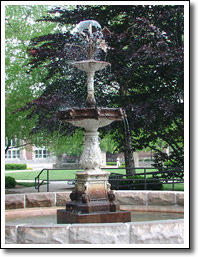Purdue University - Department of Statistics - Mathematical Statistics Option

# Mathematical Statistics OptionThe Mathematical Statistics option is a rigorous program that leads to a double major in statistics and mathematics. It prepares students for graduate work in both applied statistics and mathematical statistics or a quantitative field. Students should consider additional courses in applied statistics. Students are strongly encouraged to elect CS 15900, CS 17700 or equivalent in partial fulfillment of the laboratory science requirement or computing requirement. MA 30100 is encouraged for most students as preparation for MA 34100.

Purdue Department of Statistics, 150 N. University St, West Lafayette, IN 47907

Phone: (765) 494-6030, Fax: (765) 494-0558